# How to calculate inverse of log  base 10 in Microsoft excel

In this tutorial we will learn how to Calculate Inverse of log  base 10 in Microsoft excel. The inverse of log base 10, also known as the exponential function with base 10, is a mathematical function that calculates the power to which a base of 10 must be raised to produce a given number. In mathematical notation, it can be represented as 10^x, where x is the logarithmic value whose inverse you want to calculate.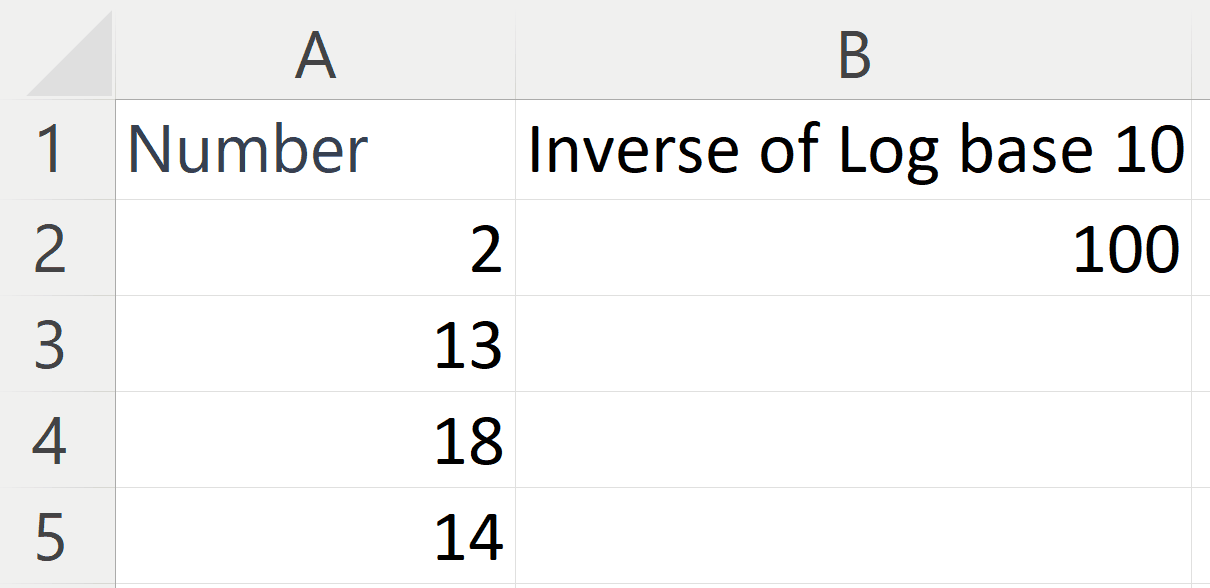Microsoft Excel is a spreadsheet software developed by Microsoft Corporation. It is a powerful tool used for organizing, analyzing and manipulating data. With Excel, you can create and edit spreadsheets, perform complex calculations, create charts and graphs, and even automate tasks using macros. Excel provides a user-friendly interface with a variety of built-in functions and features that make it easy to work with data. You can import data from a variety of sources, including other spreadsheet programs and databases, and then use the data to create pivot tables, perform statistical analysis, and generate charts and graphs to help communicate insights.

### Step 1 – Select a Blank Cell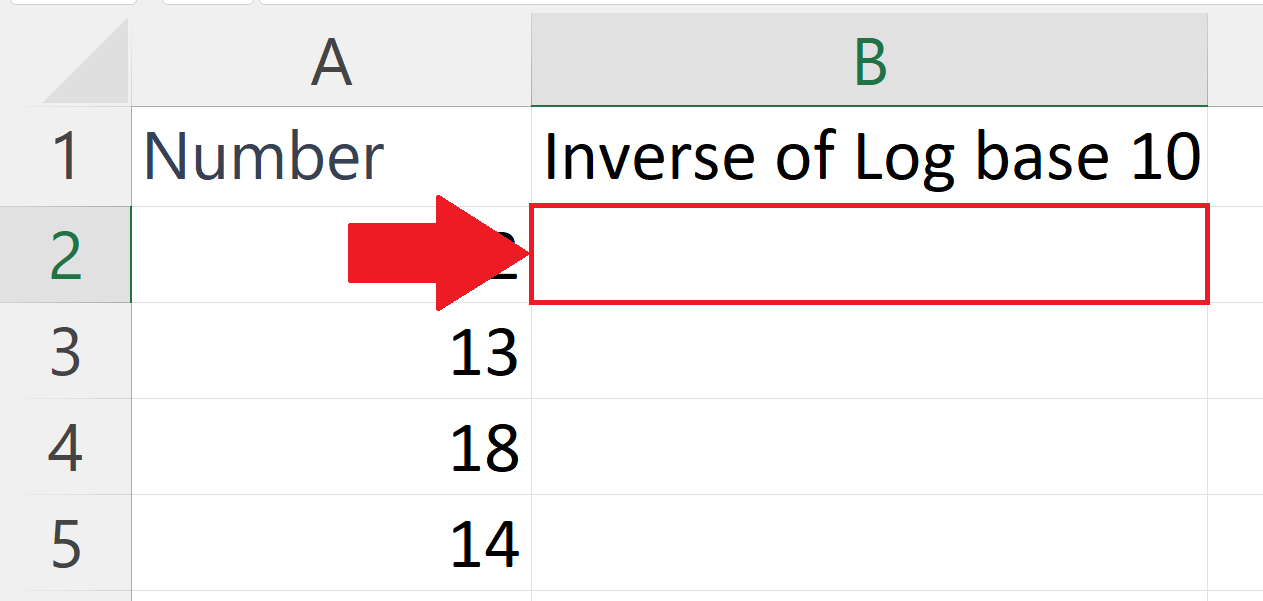– Select a blank cell where you want to calculate the inverse of log base 10.

### Step 2 – Place an Equals Sign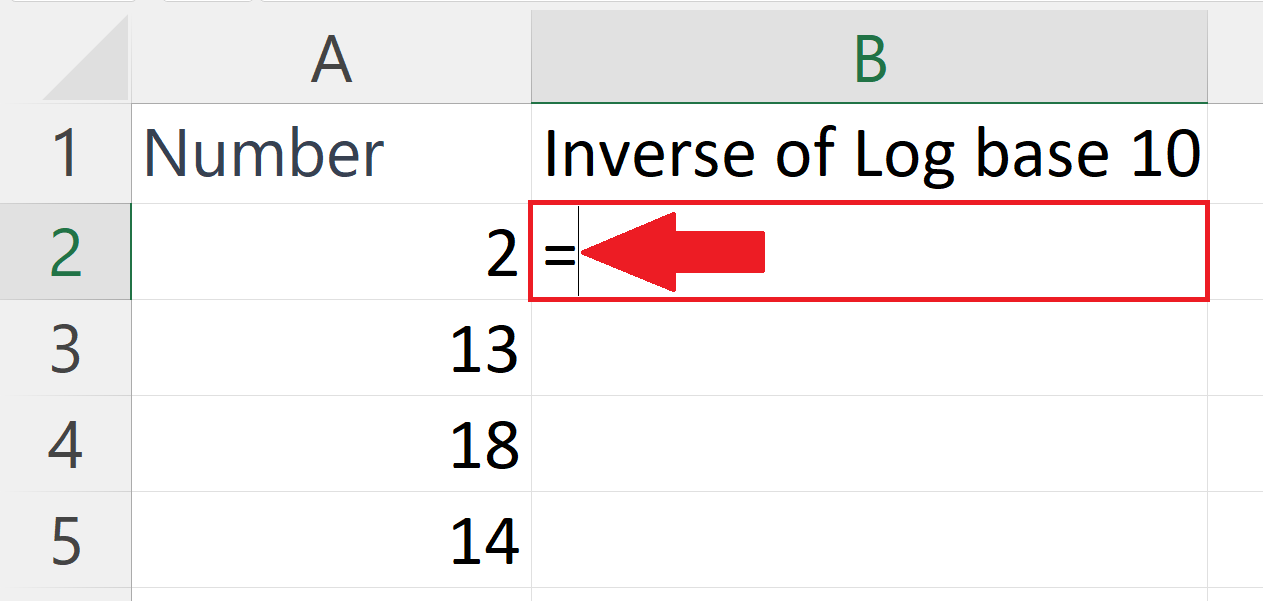– Place an Equals sign ( = ) in the blank cell.

### Step 3 – Enter the formula for inverse of log base 10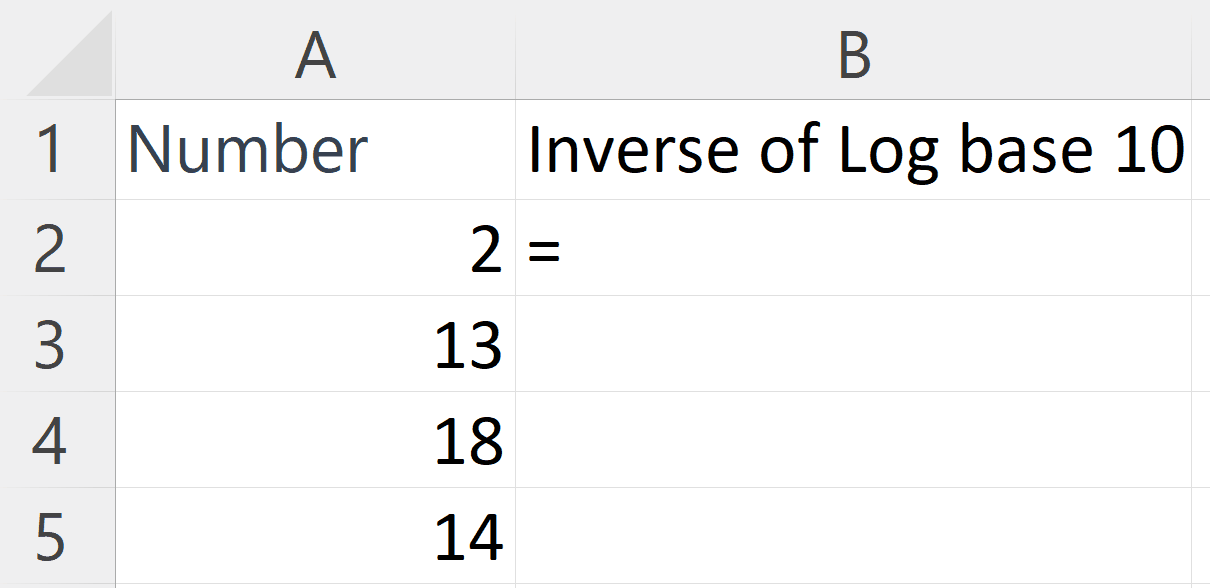– Enter the formula for inverse of log base 10 i.e.
10^( A2)
– Where the argument 10 is the base and the second argument i.e. A2 represents the address of the cell containing the number of which you want to calculate the inverse of log base 10.

### Step 4 – Press the Enter key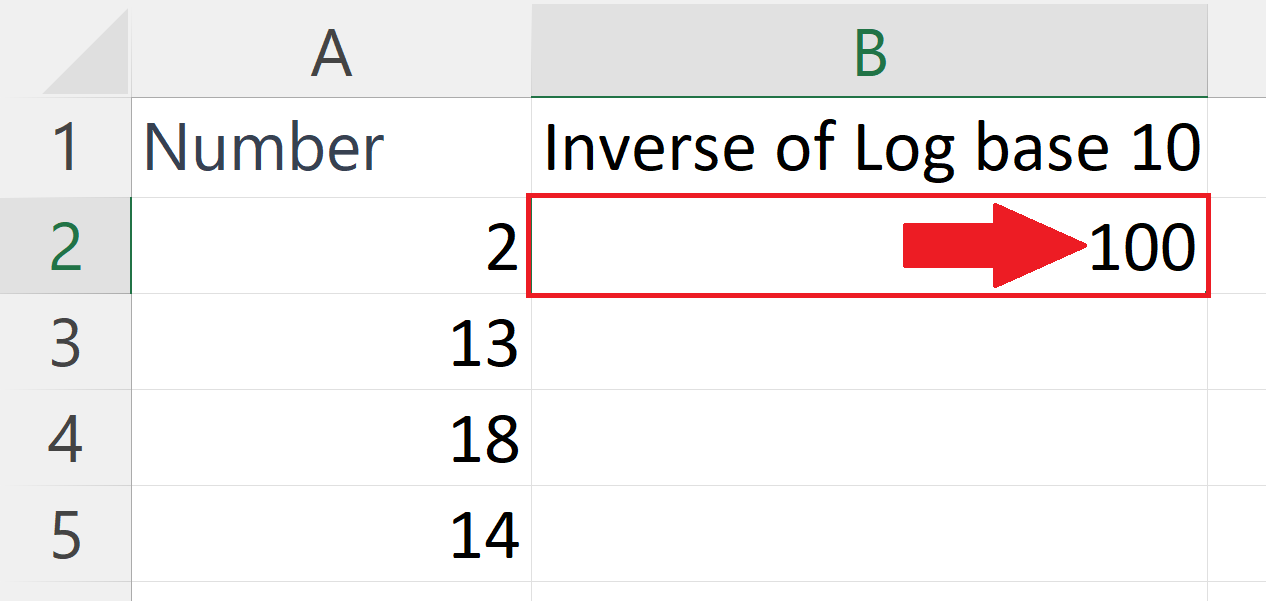– Press the Enter key to calculate the inverse of log base 10.

### Step 5 – Apply the formula on each row– Use the “Handle Select” and “Drag and Drop” method to apply the formula on each row.